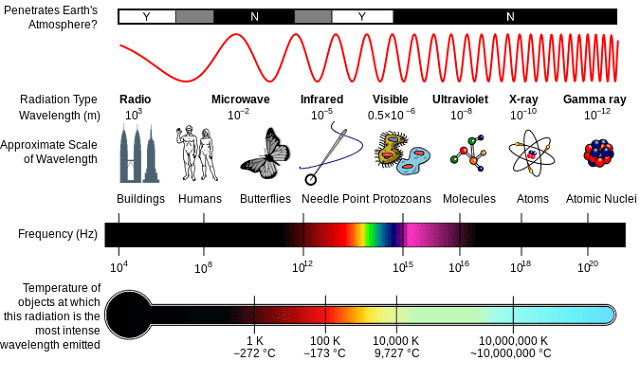# Which part of the electromagnetic spectrum has the shortest wavelength?

Q. Which part of the electromagnetic spectrum has the shortest wavelength?

The electromagnetic waves with shortest wavelengths are called gamma rays.
The electromagnetic spectrum is the range of frequencies (the spectrum) of electromagnetic radiation and their respective wavelengths and photon energies.The entire electromagnetic spectrum, from the lowest to the highest frequency (longest to shortest wavelength), includes all radio waves (e.g., commercial radio and television, microwaves, radar), infrared radiation, visible light, ultraviolet radiation, X-rays, and gamma rays.

The electromagnetic spectrum covers electromagnetic waves with frequencies ranging from below one hertz to above 1025 hertz, corresponding to wavelengths from thousands of kilometers down to a fraction of the size of an atomic nucleus. This frequency range is divided into separate bands, and the electromagnetic waves within each frequency band are called by different names; beginning at the low frequency (long wavelength) end of the spectrum these are: radio waves, microwaves, infrared, visible light, ultraviolet, X-rays, and gamma rays at the high-frequency (short wavelength) end.

So the shortest wavelength wave of the electromagnetic spectrum are gamma rays. Radio waves, on the other hand, have the lowest energies, longest wavelengths, and lowest frequencies of any type of EM radiation.

Q. Which type of electromagnetic radiation has the longest wavelength?

Q. Which electromagnetic radiation has longest wavelength?
• Radio waves. has the longest wavelength and shortest frequency.
• Microwaves. has the 2nd longest wavelength.
• Infrared radiation. has the 3rd longest wavelength.
• Visible light. has the 4th longest wavelength.
• Ultraviolet light. 5th longest wavelength`
• X rays. has the 6th longest wavelength.
• Gamma rays.has the lowest amount of wavelength but the highest frequency anomg EM Radiation

Longest Wavelength & Shortest Frequency wave are Radio Waves
Shortest Wavelength & Highest Frequency waves are Gamma RaysThanks for reading Which part of the electromagnetic spectrum has the shortest wavelength?

←Previous
« Prev Post
Next→
Next Post »